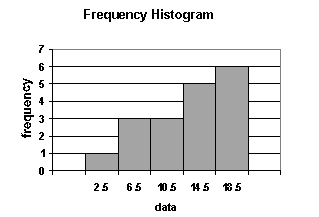### CFA Practice Question

There are 985 practice questions for this topic.

### CFA Practice Question

Use the following frequency histogram to answer the question:Which set of data is represented by the histogram?

A. 1, 3, 3, 3, 3, 3, 3, 5, 5, 5, 5, 5, 6, 6, 6, 6, 6, 6
B. 4, 6, 7, 8, 9, 10, 10, 13, 13, 13, 14, 16, 17, 17, 18, 19, 20, 21
C. 1, 5, 6, 7, 9, 9, 10, 13, 13, 14, 14, 15, 15, 17, 18, 19, 20, 20
D. 3, 5, 5, 8, 9, 11, 12, 13, 13, 14, 15, 15, 17, 17, 17, 18, 18, 20

User Comment
lavender Sorry! can anyone explain to me why the answer is D instead of B. Thanks a lot.
newsky the numbers in the 16.5 to 20.5 intervel is 6, B just has 5
nic306 I think this question is flawed. D also has 6 counts of numbers between 12.5 to 16.5 when the histrogram shows 5.
vincenthuang the class boundries are .5-4.5, 4.5-8.5, 8.5-12.5, 12.5-16.5, 16.5-20.5, D has 6 counts between 12.5-16.5, not 5 counts. So this question is flawed
samal agree with vincenthuang about class boundries, but D has 5 counts between 12.5 and 16.5! this question is correct.
bublik It's simple and it's right. The histrogram tells you that there is exactly one number in the "0.5 to 4.5" interval. That eliminates option "A". There are 6 numbers in the "16.5 to 20.5" interval. That eliminates choices "B" (5 numbers fall in specified interval) and "C" (5 numbers fall in specified interval). The remaining option "D" has exactly six numbers in the "16.5 to 20.5" interval, exactly as specified by the histogram.
stefdunk B has the following numbers in the "16.5 to 20.5" interval: 17, 17, 18, 19, 20, 21
for a total of 6
limpus stefdunk, please tell me how 21 falls into the "16.5 to 20.5" bracket
gord I think this histogram is flawed? It is missing a frequency of 1 bar for a 22.5 rating to account for the 21 observation.
chuong What is the meaning of 2.5, 6.5 ... what is the exact interval of this question. I counted 18 obserations from the histogram
sarath Important is to determine the class intervals..
2.5, 6.5, 10.5, 14.5, 18.5 are the interval midpoints.
The "interval gap" = 4 (get this subtracting any two adjacent midpoints)
You can get the classes by adding 1/2 of the "interval gap" to both sides of the midpoint to give:
0.5 - 4.5 : 1 (i.e. 3)
4.5 - 8.5 : 3 (i.e. 5,5,8)
8.5 - 12.5: 3 (i.e. 9,11,12)
12.5- 16.5: 5 (i.e. 13,13,14,15,15)
16.5 -20.5: 6 (i.e. 17,17,17,18,18,20)
willeen Many thanks akanimo, great explanation!
surob Wow, that is simple, but good question!!!
vadimmuchnik Akanimo - the best explanation! thanks!
coolnan Thanks-akanimo! This is a basic yet a very good question
mallang dat was a tough 1ne.
mwali Akanimo
I have followed upto getting the classess but going further am lost . Please explain how the answer is D.
mrushdi Akanimo from Japan is the man.
Beret Yeah, the "interval midpoints" remark enlightened me as well. Thanks Akanimo
Mirlin01 sum the frequency. it sums to 18 observations (1+3+3+5+6=18). the only dataset having 18 observations is data set A. thus i would select A as correct.
Mariecfa Merlin01: All A,B,C, and D have 18 observations. Akanimo is correct that it is D.
Matt26 fantastic
ChrisYung88 Akanimo how do you get the classes again?
fsammari I don't understand how dir they get the classes
2014 Akamino is talk of the town across world ...
Thanks simple easy u made for us
bidisha you get classes by subtracting 1/2 from both side of each number like akanimo said. I arranged it like this, so:

total distance between each interval is 4. (so 6.5-2.5=4, 10.5-6.5=4 etc)

Now what does 4 mean? It means on each side is 2. 2 on the left side and 2 on the right side. So for the first one 2.5 you subtract 2 from 2.5=0.5 and then add 2 to 2.5=4.5
So classes:
0.5-(2.5)-4.5-(6.5)-8.5-(10.5)-12.5-(14.5)-16.5-(18.5)

Last step is just counting:
0.5-2.5-4.5=1
6.5-8.5=3
10.5-12.5=3
14.5-16.5=5 etc

Thanks Akanimo you made it easy